# Managing Engineering and Technology Study Set 1

## Quiz 11 : Planning Production ActivityStudy FlashcardsLooking for Management Homework Help?

## Quiz 11 :Planning Production Activity

Question TypeSetup for a stamping operation required a time-consuming fixture installation and testing that took 4 hours each time a different part was to be produced; typically, 12 hours' production was made for inventory of a given stamping before the machine was stopped to permit setup for a new part. After careful process analysis, fixtures and transfer methods were revised to permit setup in 15 minutes. Discuss the implications for this change on ( a ) optimum batch size, ( b ) order frequency, and ( c ) machine and labor productivity.
Free
Essay

(a)
If the setup time is reduced from 4 hours to 15 minutes, then the Economic Order Quantity (EOQ) optimum batch size will decrease. The company will be able to produce smaller lot sizes and thus have to hold fewer inventories.
(b)
Smaller EOQ batch sizes will imply that the order frequency for production will increase. Rather than making longer orders and building up more inventories, the company can make more frequent smaller orders and reduce inventory levels.
(c)
Reduced setup time means that the machine and labor has to spend more time producing goods, rather than being re-tooled for making a different product. This will increase both the labor and machine productivity since more time is spent on value-adding activities.

Tags
Choose question tagA plant is beginning production of a light-alloy product and finds that it takes 400 hours to produce the first item. How many hours should it take to produce each of the following: ( a ) the second item; ( b ) the eighth item; ( c ) the 37th item? ( Hint: Refer to Table) Table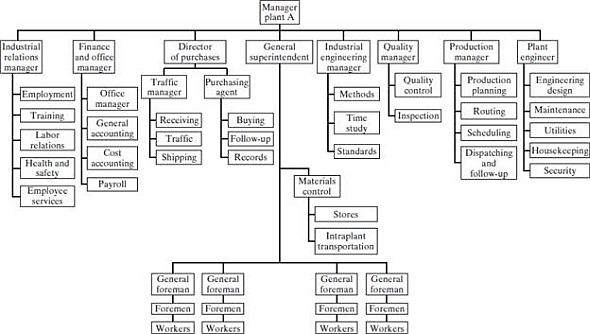Free
Essay

Calculate the time required to produce given number of product units for the plant, using given details:
Time required for production of 1 unit = 400 hours
Percent learning rate for light alloy product = 80 percent
(a)
Solve this problem using the learning curve formula shown below: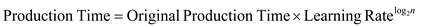… (1)
The learning rate for the light-alloy manufacturing industry is about 80 percent, the original production time is 400 hours, and n for the second item is 2.
Now putting the above values in formula (1), obtain the following results:Hence, the total production time for second item would be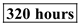(b)
Calculate the production time for eighth item by putting n= 8 in the given formula (1), as shown below:Thus, total production time for the eighth item is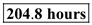.
(c)
Calculate production time for the 37th item by putting n= 37 in formula (1), as shown below:Therefore, total production time for the 37th item would be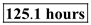Tags
Choose question tagThe first two units of a product cost a total of $9,000 to produce. If you believe an 80 percent learning curve applies, how much would you expect the fourth unit to cost? Free Essay Answer: Answer: Calculate the time required to produce fourth unit of a product, using given details: Cost required for production of 2 units =$ 9000
Percent learning rate for the product = 80 percent
(a)
Solve this problem using the learning curve formula shown below: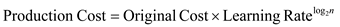… (1)
The learning rate is 80 percent and n for the fourth item is 4. Calculate the cost of the first unit using the following expression:Use the fact that cost for the first two units was $9000, to calculate the cost involved in production of the first unit.Now, calculate the cost of fourth unit using equation (1), as shown below:Thus, the cost involved in manufacturing of fourth unit would be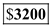. Tags Choose question tagWhat subjects will be important in the education of the manufacturing engineer for the twenty-first century? Essay Answer: Tags Choose question tagIf it costs$2.00 per unit to store an item for one year and $40.00 setup cost every time you produce a lot, and you use 1,000 units per year, how many lots of what size should be manufactured each year? ( b ) How would your answer change if the setup cost can be reduced to$10.00?
Essay
Tags
Choose question tagDiscuss how the management functions of planning, organizing, leading, and controlling relate to the production planning process.
Essay
Tags
Choose question tagList some of the characteristics that make the Dunn and Savastano Orthodontics business compete with other practices.
Essay
Tags
Choose question tagDiscuss some of the factors that would be most important in selecting a site for ( a ) a Portland cement plant, ( b ) a research "think tank," and ( c ) a shoe factory.
Essay
Tags
Choose question tagDistinguish between MRP and MRP II.
Essay
Tags
Choose question tagWhat is the relationship between FMS and the group technology concept introduced earlier in the chapter?
Essay
Tags
Choose question tagDescribe how the kanban is used in the just-in-time production system.
Essay
Tags
Choose question tagWhat are some of the reasons for using a flexible manufacturing system?
EssayA production plant with fixed costs of $300,000 produces a product with variable costs of$40.00 per unit and sells them at $100 each. What is the break even quantity and cost? Illustrate with a break-even chart. Essay Answer: Tags Choose question tagWhat are some of the positions that engineers fill in a large manufacturing plant? Essay Answer: Tags Choose question tagWhy is a vigorous production capability so important to the United States in the "postindustrial society"? Essay Answer: Tags Choose question tagDistinguish between ( a ) product layout, ( b ) process layout, and ( c ) group technology Essay Answer: Tags Choose question tagA machine tool salesperson offers the plant of Question 11-2 equipment that would increase their fixed cost by$180,000, but reduce their variable cost from $40.00 to$25.00. Should the plant accept this suggestion if they can sell their entire plant capacity of 10,000 units per year at \$100 each? Illustrate by modifying the break-even chart of Question 11 2.